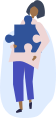Chemistry Homework Help

Get step-by-step solutions from expert tutors.

## How it works?Type, take a picture, or paste your question with all necessary details to ensure high-quality responses.### Get an answer in less than 24hrs

Once our tutors receive the question, they'll begin. You'll get an email once the solution is ready.### Get Notified

We will send you email and text notification to let you know when your question is answered.

### Browse Homework Solutions

12,704 solutions

Le Chatelier's Principle

#### Q. Oxygen and ozone are allowed to come to equilibrium in the exothermic reaction: 2O3(g) ⇌ 3 O2(g). Which change will increase the numerical value of the equilibrium constant, K?

Solved • Dec 13, 2019

Le Chatelier's Principle

#### Q. Consider this reaction at equilibrium. 2 SO2(g) + O2(g) ⇌ 2 SO3(g) ΔH=-198 kJ. Which change would cause an increase in the SO3/SO2 mole ratio? (A) adding a catalyst (B) removing O2(g) (C) decreasing the temperature (D) decreasing the pressure

Solved • Dec 13, 2019

Le Chatelier's Principle

#### Q. This reaction reaches equilibrium in a closed container. What happens if the volume of the container is decreased art constant temperature? Ch4(g) + H2O(g) ⇌ CO(g) + 2 H2(g)

Solved • Dec 13, 2019

Chemical Equilibrium

#### Q. The value of an equilibrium constant can be used to predict each of these except one.

Solved • Dec 13, 2019

Equilibrium Expressions

#### Q. What is the Kc for the reaction? P4(s) + 6 Cl2(g) ⇌ 4 PCl3(g)

Solved • Dec 13, 2019

Equilibrium Expressions

#### Q. What is the Kp for this reaction? 2 SO3(g) ⇌2 SO2(g) + O2(g)

Solved • Dec 13, 2019

Equilibrium Expressions

#### Q. What is the equilibrium expression for this reaction: 2S(s) + O2(g) ⇌ 2CO(g)

Solved • Dec 13, 2019